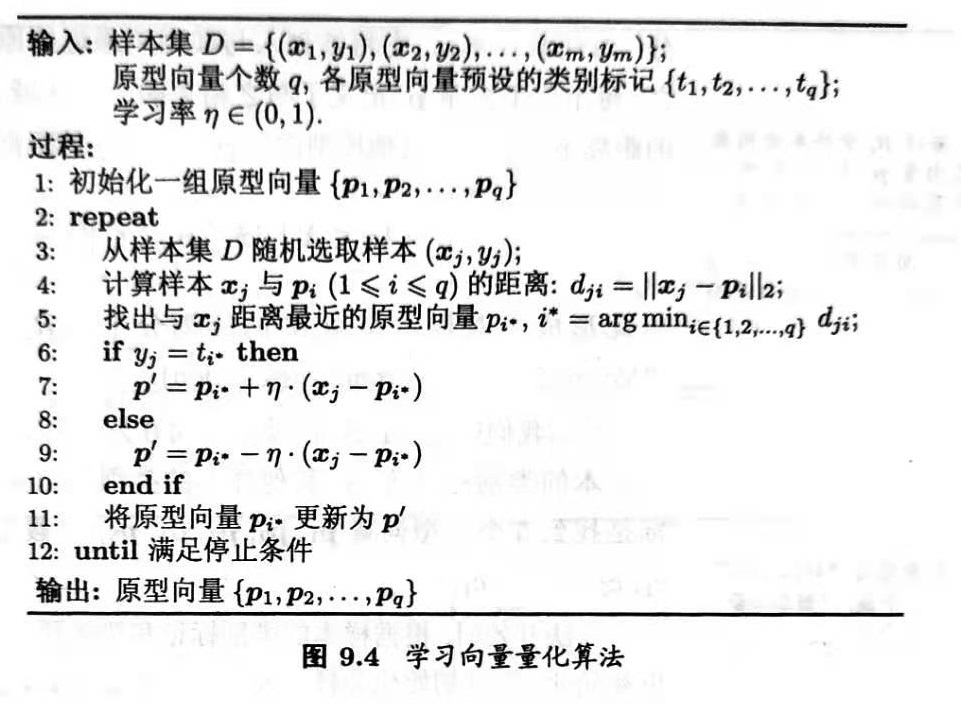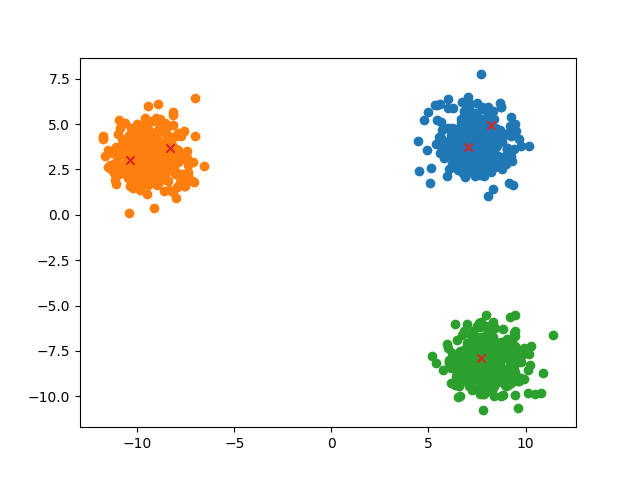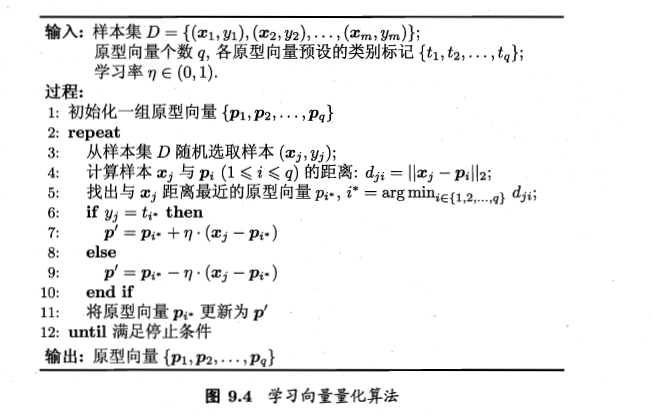• 学习向量量化算法1. 聚类算法家族族谱2. 学习向量量化3. 数据准备4. 算法流程 1. 聚类算法家族族谱 这篇文章主要介绍了：聚类算法的特点，样本间距离，簇之间距离的计算方法以及衡量聚类算法性能的算法。 2. 学习...
学习向量量化算法1. 聚类算法家族族谱2. 学习向量量化3. 数据准备4. 算法流程5. 代码实现6. 参考文献
1. 聚类算法家族族谱
这篇文章主要介绍了：聚类算法的特点，样本间距离，簇之间距离的计算方法以及衡量聚类算法性能的算法。

2. 学习向量量化
学习向量量化（Learning Vector Quantization, LVQ）同KMeans算法类似，也是要找一个具有代表性的值代表某一类别。但是其输入数据是要求有类别标签的。如果说KMeans聚类算法是样本空间中的样本点自发聚集，然后选出一个最有代表性的值的话，那么LVQ算法就是通过不断“调教“某一个值，使其最后能代表整个簇。
通俗的说：KMeans算法是不断的通过海选选择出一个最具有代表性的值，而LVQ算法更像是一个菜鸡的成长史，从一个普通的值，最后成长为能够代表一个类的值。
3. 数据准备
给定样本集$D=\{(\boldsymbol{x_1}, y_1),(\boldsymbol{x_2}, y_2),...,(\boldsymbol{x_m}, y_m) \}$其中，每个样本$\boldsymbol{x_j}$是有$n$个属性的特征向量$(x_{j1}, x_{j2},..., x_{jn})$，$y_j$是第$j$个样本的类别标记。
假设最后学习的原型向量维度为$d$，共有$q$个，记为：$\{\boldsymbol{p_1}, \boldsymbol{p_2}, ..., \boldsymbol{p_q}\}$，其中每个原型向量代表一个聚类簇，簇标记$t_i \in Y$。
4. 算法流程
学习向量的学习流程如下：学习向量完成后，便可通过计算各个点与学习向量的距离来判断该点属于哪个簇。

5. 代码实现
使用的数据为西瓜数据4.0
代码地址
import numpy as np
import pandas as pd
from random import randint
from Cluster.Distance import DistMinkov
from Cluster.Visulization import clusterClubs

'''
读取数据
:param filename: 文件名
:return: 文件中的数据
'''
return data

class LVQ(object):
def __init__(self, dist_parameter, lr=0.1, cluster_num=5):
'''
初始化 LVQ 类
:param dist_parameter: 计算距离函数的相关信息
:param lr: 学习率
:param cluster_num: 聚类簇的数量
'''
self.lr = lr
self.cluster_num = cluster_num
self.nums = 0
self.features = 0
self.distant_func = dist_parameter['function']
self.parameter = dist_parameter['parameter']
self.cluster = {}

def __initArgs__(self, Xdata, Ydata):
'''
初始化参数：学习向量
:param Xdata: 特征值
:param Ydata: 标签值
:return: 初始化完成的特征向量
'''
self.nums, self.features = Xdata.shape
# 选中的初始化学习向量
index_arr = np.random.randint(1, self.nums, size=self.cluster_num)
for key, index in enumerate(index_arr):
self.cluster[key] = {
'mean_vec': Xdata[index],
'label': Ydata[index],
'data': np.zeros((1, self.features))
}

def fit(self, Xdata, Ydata, iterations=100):
'''
拟合数据
:param Xdata: 特征数据
:param Ydata: 标签数据
:param iterations: 迭代次数
:return: 聚类结果
'''
self.__initArgs__(Xdata, Ydata)
for iters in range(iterations):
print('iteration:', iters)
# 从样本空间中随机选一个作为训练数据
train_index = randint(0, self.nums-1)
Xtrain = Xdata[train_index]
Ytrain = Ydata[train_index]

min_dist = float(np.inf)
update_index = -1
# 计算该点到所有簇心的距离，取最短距离，记录学习向量的索引值
for lv_index in self.cluster.keys():
dist = self.distant_func(
Xtrain, self.cluster[lv_index]['mean_vec'],
p=self.parameter
)
if min_dist > dist:
min_dist = dist
update_index = lv_index
# 更新参数
self.update_learning_vector(
Xtrain, Ytrain, update_index
)

return self.cluster

def update_learning_vector(self, Xtrain, Ytrain, update_index):
'''
更新权重
:param Xtrain: 训练数据的特征值
:param Ytrain: 训练数据的标签值
:param update_index: 需要更新的学习向量的索引
:return:
'''
update_feature = self.cluster[update_index]['mean_vec']
update_label = self.cluster[update_index]['label']

if Ytrain == update_label:
update_feature += self.lr * (Xtrain - update_feature)
else:
update_feature -= self.lr * (Xtrain - update_feature)
self.cluster[update_index]['mean_vec'] = update_feature

def predict(self, Xdata):
'''
对数据进行聚类
:param Xdata: 数据特征值
:return: 聚类结果
'''
for idata in Xdata:
min_dist = float(np.inf)
cluster_name = 0
# 对于每个数据选出距离最近的簇
for icluster in self.cluster.keys():
dist = DistMinkov(
self.cluster[icluster]['mean_vec'],
idata,
p=2
)
if min_dist > dist:
min_dist = dist
cluster_name = icluster

self.cluster[cluster_name]['data'] = np.r_[
self.cluster[cluster_name]['data'],
np.array([idata])
]
return self.cluster

def main():
filename = 'data/WaterMelon4.txt'
Xdata = data[['density', 'sugercontent']].values
Ydata = data['label'].values
dist_parameter = {
'function': DistMinkov,
'parameter': 2
}
clf = LVQ(
dist_parameter,
lr=0.1
)
clf.fit(
Xdata,
Ydata,
400
)
cluster = clf.predict(Xdata)

clusterClubs(cluster)
if __name__ == '__main__':
main()

距离计算公式
def DistMinkov(xi, xj, p=2):
'''
计算两个向量的曼哈顿距离(第一范数)
:param xi: 向量xi, <1*n>
:param xj: 向量xj, <1*n>
:return: xi与xj之间的曼哈顿距离
'''
x = xi - xj
data = np.apply_along_axis(np.power, 0, x, p)
sum_data = np.sum(data)
return pow(sum_data, 1/p)


6. 参考文献

《西瓜书》


展开全文聚类
• Ipynb源文件，学习向量量化Python实现，使用鸢尾花数据集
• 学习向量量化 “学习向量量化”(Learning Vector Quantization，简称LVQ)是一种利用监督信息辅助的聚类算法，像K-Meas算法一样也是通过调整一组类似于质心的点来进行聚类。 算法描述 假设给定样本D={(X1,Y1),...

学习向量量化

“学习向量量化”(Learning Vector Quantization，简称LVQ)是一种利用监督信息辅助的聚类算法，像K-Meas算法一样也是通过调整一组类似于质心的点来进行聚类。

算法描述

假设给定样本D={(X1,Y1),(X2,Y2),…,(Xm,Ym)},X表示样本的属性，Y表示样本的类别标记。LVQ目的是学习一组n维原型向量{P1,P2,…Pq}，当样本点距离这组原型向量的某个分向量距离最近时那么它的类别标记应当与这个原型分向量一致。原型向量的训练过程和K-Means算法大同小异，初始时通过随机一组原型向量(可以从样本点中随机提取)，当与原型向量最近的样本点类别标记与此原型向量的类别不同时，那么要调整原型向量，使得原型向量离当前的样本点远一些，相反则需要调整它离当前样本点近一些，当算法满足停止条件时(达到最大迭代次数，或原型向量更新变化很小甚至不变时)则可以返回这一组原型向量。代码实现：
生成数据集：
X=datasets.make_blobs(n_samples=1000,centers=3) #1000个样本点分为3类

初始化原型向量：
P=np.zeros((q,col)) #原型向量
for i in range(q):   #初始化原型向量
index=np.where(sample==Label[i])
choose=np.random.randint(0,len(index),1)
P[i,:]=sample[index[choose],:]

训练主体：
for i in range(1000):   #训练
choose=np.random.randint(0,row,1) #随机选取一个样本
dis=np.linalg.norm(sample[choose,:]-P,axis=1) #计算与原型向量的距离
y=dis.tolist().index(min(dis))  #获取距离最近的原型向量下标
if Label[y]==sample[choose]: #更新原型向量
P[y,:]=P[y,:]+eta*(sample[choose,:]-P[y,:])
else:
P[y,:]=P[y,:]-eta*(sample[choose,:]-P[y,:])

完整代码：
from sklearn import datasets
import matplotlib.pyplot as plt
import numpy as np
X=datasets.make_blobs(n_samples=1000,centers=3) #1000个样本点分为3类

def lvq(sample,q,Label,eta):
if q!=len(Label):
return 0
row,col=np.shape(sample) #获取样本集的规格
P=np.zeros((q,col)) #原型向量
for i in range(q):   #初始化原型向量
index=np.where(sample==Label[i])
choose=np.random.randint(0,len(index),1)
P[i,:]=sample[index[choose],:]
for i in range(1000):   #训练
choose=np.random.randint(0,row,1) #随机选取一个样本
dis=np.linalg.norm(sample[choose,:]-P,axis=1) #计算与原型向量的距离
y=dis.tolist().index(min(dis))  #获取距离最近的原型向量下标
if Label[y]==sample[choose]: #更新原型向量
P[y,:]=P[y,:]+eta*(sample[choose,:]-P[y,:])
else:
P[y,:]=P[y,:]-eta*(sample[choose,:]-P[y,:])
IDX=[]  #分类标记
for i in sample:  #以距离最近的标记为样本的类别
D=np.linalg.norm(i-P,axis=1)
y=D.tolist().index(min(D))
IDX.append(Label[y])
plot(IDX,sample,max(Label)+1,P)
return P
def plot(a,X,k,p):  #绘画板块
m=k
for j in range(m):
index=[i for i,v in enumerate(a) if v==j]
x=[]
y=[]
for k in index:
x.append(X[k])
y.append(X[k])
plt.scatter(x,y)
plt.scatter(p[:,0],p[:,1],marker='x')
plt.show()

测试代码:
lvq(X,5,[0,1,0,1,2],0.3)

array([[  7.02402226,   3.74801884],
[-10.38672182,   3.03633487],
[  8.20578187,   4.94912081],
[ -8.30953565,   3.69883677],
[  7.69178827,  -7.90175793]])展开全文聚类 算法
•研究论文
• 学习向量量化（Learning Vector Quantization，简称 LVQ）与 K 均值算法类似，也是试图找到一组原型向量来刻画聚类结构，但与一般聚类算法不同的是，LVQ 假设数据样本带有类别标记，学习过程利用样本的这些监督信息...
学习向量量化（Learning Vector Quantization，简称 LVQ）与 K 均值算法类似，也是试图找到一组原型向量来刻画聚类结构，但与一般聚类算法不同的是，LVQ 假设数据样本带有类别标记，学习过程利用样本的这些监督信息来辅助聚类。
给定样本集 $D = \{(x_1, y_1), (x_2, y_2), \cdots, (x_n, y_n)\}$，每个样本 $x_i$ 是由 m 个属性描述的特征向量 $(x_i^1, x_i^2, \cdots, x_i^m), y_i \in Y$ 是样本 $x_i$ 的类别标记。
【目标】：学得一组 m 维原型向量 $\{p_1, p_2, \cdots, p_k\}$，每个原型向量代表一个聚类簇，簇标记 $t_j \in Y$。
【算法描述】：

输入：样本集 $D = \{(x_1, y_1), (x_2, y_2), \cdots, (x_n, y_n)\}$；原型向量个数 k，各原型向量预设的类别标记 $\{t_1, t_2, \cdots, t_k\}$；学习率 $\eta \in (0, 1)$。
输出：原型向量 $\{p_1, p_2, \cdots, p_k\}$。
过程：

从样本集 D 中随机选取样本作为原型向量（初始均值向量）；
在每一轮迭代中，随机选取一个样本，并计算该样本与各个原型向量的距离，然后确定簇标记；
根据样本和原型向量的类别标记是否一致来进行相应的更新：

若簇标记相等，则将原型向量向该样本靠近；
$p' = p_{j*} + \eta (x_i - p_{j*})$
若簇标记不相等，则将原型向量远离该样本；
$p' = p_{j*} - \eta (x_i - p_{j*})$

若满足算法的停止条件（指定的迭代次数），则将当前原型向量作为最终结果返回。

在学得的一组原型向量 $\{p_1, p_2, \cdots, p_k\}$ 后，即可实现对样本空间 X 的簇划分。之后，对任意样本 x，将其划入与其距离最近的原型向量所代表的簇中。换言之，每个原型向量 $p_i$ 定义了与之相关的一个区域 $R_i$，该区域中每个样本与 $p_i$ 的距离不大于它与其他原型向量 $p_{i'}(i'\neq i)$ 的距离，即
$R_i = \{x \in \chi | ||x-p_i||_2 \leq ||x-p_{i'}||_2, i' \neq i \}$
由此形成了对样本空间 X 的簇划分 $\{R_1, R_2, \cdots, R_k\}$，该划分通常称为 “Voronoi 剖分”（Voronoi tessellation）。若将 Ri 中样本全用原型向量 pi 表示。则可实现数据的“有损压缩”（lossy compression），这称为“向量量化”（vector quantization）；LVQ 由此而得名。
案例说明
构造一个数据集用以说明 LVQ 的执行过程，数据集内容如下：
[3, 4, 1]
[3, 3, 1]
[1, 2, 0]
[1, 1, 0]
[2, 1, 0]

前两列为样本的特征数据 X，最后一列为样本的标签值 Y。假设，此时我们将上述数据集划分为两个簇，并随机挑选 x1 和 x3 作为初始原型向量 p1 和 p2，学习率 $\eta = 0.1$。
在第一轮迭代中随机选取的样本 x2，分别计算 x2 与 p1 和 p2 的距离为 1 和 $\sqrt{5}$，x2 与 p1 距离更近且具有相同的标签值。
$p_1' = p_1 + \eta \cdot (x_1-p_1) = (3; 4) + 0.1 * ((3; 3) - (3; 4)) = (3; 3.9)$
将 p1 更新为 p1’ 之后，不断重复上述过程，直到满足终止条件。
【存在问题】：若挑选 x1 和 x2 或 x3 和 x4 作为初始簇，那么我们最后得到的两个原型向量的标签值都是相同的，例如都为 0。这样就会导致无论输入的样本与哪个原型向量更接近，其标签值最终都为 0，这显然不是我们希望得到的结果。因此在挑选初始原型向量时需要将当前样本集的所有标签值都囊括在初始原型向量集中。
代码实现
【所需包】：

NumPy

【随机挑选初始均值向量函数】：随机确定起始位置，然后根据样本集长度和挑选数量计算出步长。这么做可以避免挑选出重复值，以及尽可能地从样本集各个区域挑选数据，而不是聚集在某一区域。
def random_select(dataset, count):
# 获取样本集长度
length = dataset.shape
# 计算步长和初始位置
step, start = length // count, np.random.randint(length)
data_select = []
# 按照起始位置和步长挑选数据
for i in range(count):
data_select.append(dataset[start])
start += step
start = start % length
return data_select

【LVP 函数实现】：

随机挑选指定数量的原型向量。

prototypes = random_select(dataset, k)


开始迭代。

for i in range(n_iters):
# ...


迭代过程中，每次随机从样本集中选择一个样本，判断该样本所属的簇。

data = dataset[numpy.random.randint(length)]
min_dist = numpy.inf
cluster_index = -1
for j in range(k):
dist = numpy.linalg.norm(data[:2] - prototypes[j, :2], 2)**2
if dist < min_dist:
min_dist = dist
cluster_index = j


接着，判断当前样本的标签类别是否和所属簇的类别标签是否相同。根据不同的情况执行相应的操作。

if data == prototypes[cluster_index, 2]:
prototypes[cluster_index, :2] += learning_rate * (data[:2] - prototypes[cluster_index, :2])
else:
prototypes[cluster_index, :2] -= learning_rate * (data[:2] - prototypes[cluster_index, :2])


迭代结束后，返回原型向量。

return prototypes

【完整代码】：传送门
def lvp(dataset, p, n_iters=100, learning_rate=1):
length = dataset.shape
prototypes = random_select(dataset, p)

for i in range(n_iters):
# 随机挑选样本
data_random = dataset[np.random.randint(length)]
min_dist = np.inf
cluster_index = -1
# 判断当前样本所属的簇
for j in range(p):
dist = np.linalg.norm(data_random[:2] - prototypes[j, :2], 2)
if dist < min_dist:
min_dist = dist
cluster_index = j
if data_random == prototypes[cluster_index, 2]:
prototypes[cluster_index, :2] += learning_rate * min_dist
else:
prototypes[cluster_index, :2] -= learning_rate * min_dist
return prototypes

参考

《机器学习》周志华


展开全文• 学习向量量化 说明：与其总结不清楚，不如给出参考资料与代码供大家学习讨论。 参考资料： 1.《机器学习》周志华P205页 2. 哔哩哔哩 https://www.bilibili.com/video/BV1fi4y1K7Ke?p=3 3. link 1.算法步骤 2.matlab...
学习向量量化
说明：与其总结不清楚，不如给出参考资料与代码供大家学习讨论。
参考资料：
1.《机器学习》周志华P205页
2. 哔哩哔哩 https://www.bilibili.com/video/BV1fi4y1K7Ke?p=3
1.算法步骤2.matlab代码
function p=mylvq(data,label,q,learn_rate,maxIter)
%mylvq generate q Prototype vector.
%   Input arguments:
%       label:  label of data;
%       data :  m-by-n matrix of n m-dimensional data points.
%       q    :  the number of Prototype vector
%       learn_rate: learning rate. Default 0.1
%       maxIter:    Maximum number of iterations. Default 1e4
%   Output arguments:
%       p: Prototype vector

%-------------------------------------------------------------------------------
%  ChangChun University of Science and Technology,ChangChun,130022,China
%  Contact author at 907353999@qq.com
%-------------------------------------------------------------------------------

if nargin<5 && 4<=nargin
maxIter=1e4 ;
elseif nargin<4 && 3<=nargin
maxIter=1e4 ;
learn_rate=0.1;
elseif nargin<3
error('Insufficient input parameters!!!')
end

%% step0 initialize a set of Prototype vector
m = size(data,1); % dimension of points
n = size(data,2); % number of points
labelnum=length(q);
p=[];
p_label=[];
for i=1:labelnum
tempdata=data(:,label==i);
% choose q unique random indices between 1 and n (number of points)
randIdx = randperm(size(tempdata,2),q(i));
% initial
p=[p,tempdata(:,randIdx)];
p_label=[p_label,repmat(i,1,q(i))];
end

%% step1 repeat
newp = p;
iter=0;
while 1
idxS = randperm(n,1);       %idxS---Index of Sample point
% init distance array dist
distance = zeros(1,sum(q));
% compute distance to each Prototype vector
for idxP=1:sum(q)
distance(idxP) = norm(data(:,idxS)-p(:,idxP));
end
% find index of closest Prototype vector
[~, nearestP] = min(distance);
if label(idxS)==p_label(nearestP)
newp(:,nearestP)=p(:,nearestP)+learn_rate*(data(:,idxS)-p(:,nearestP));
else
newp(:,nearestP)=p(:,nearestP)-learn_rate*(data(:,idxS)-p(:,nearestP));
end

% Checking for stopping criterion1: Meet maximum number of iterations
iter = iter + 1;
if iter > maxIter
break;
end

% Checking for stopping criterion2: Prototype vector do not chnage anymore
if newp==p
break;
end
% update previous cluster clusterPrev
p = newp;

end

% for reference: print number of iterations
fprintf('mykmeans.m used %d iterations of changing centroids.\n',iter);




展开全文• 基于学习向量量化神经网络的人脸朝向识别方法_冯洁琼.caj
• 在竞争网络结构的基础上，学习向量化（learning vector quantization，LVQ）网络被提出来，融合竞争学习思想和有监督学习算法的特点，通过教师信号对输入样本的分配类别进行规定，从而克服自组织网络...向量量化的思...
• 一种改进向量量化算法的图象压缩研究 LBG向量算法的改进 增加向量的维数
• 根据码书生成过程，可将传统向量量化方法分为7类：树形向量量化、直和向量量化、迪卡尔积向量量化、格子向量量化、基于分类的向量量化、反馈向量量化以及模糊向量量化。在过去10年中，基于向量量化的近似近邻搜索...
• 学习向量量化（LVQ） 是一种无模型的数据点聚类方法。可以用来执行分类任务。 （他很难搞清楚反应变量y和预测器x之间存在什么样的联系。因而在许多现实的场景中，它是作为一种黑箱方法来加以应用的。） 步骤： ...数据挖掘 机器学习 python
• 学习向量量化(Learning Vector Quantization) 学习向量量化(Learning Vector Quantization,简称LVQ)属于原型聚类，即试图找到一组原型向量来聚类，每个原型向量代表一个簇，将空间划分为若干个簇，从而对于任意的...机器学习
• 针对现有煤岩识别方法适用范围小、识别正确率低等问题,采用图像分块离散...采用学习向量量化神经网络进行煤岩识别,2种特征提取方式的识别准确率均为96.67%,比Haar小波方法提高了3.3%,比Daubechies小波方法提高了5.8%。
• 一文详解，原型聚类只学习向量量化算法背后原理。码字不易，喜欢请点赞，谢谢！！！ 一、学习向量量化简介 前面的文章中，介绍过k-means和层次聚类这两种聚类方法，今天介绍一种新的聚类算法，即学习向量量化...原理
• 一、学习向量量化  LVQ神经网络是一种混合网络，通过有监督及无监督的学习来形成分类。  在LVQ网络中，第一层的每个神经元都制定给某给类，常常几个神经元被制定给同一类，每类再被指定给第二层的一个神经元。第...
• 利用多波束测深系统获取的反向散射强度数据，应用学习向量量化(learning vector quantization，LVQ)神经网络分类方法实现了对海底砂、砾石和基岩等底质类型的快速、有效的识别。通过比较，证明了该方法能较好地区分...
• 学习向量量化简介 ：学习向量量化是一种聚类算法，我自己感觉这应该是一种监督学习算法吧，这种算法属于原型聚类，找到初始原型来刻画聚类结构，与一般聚类算法不同的是，(LVQ)这种算法数据样本带有监督信息标记算法...机器学习
• 学习向量量化与K均值算法类似，但是K均值算法是在原数据没有类别标记的情况下，是一种无监督算法，而学习向量量化算法是在数据集类别标签的这些监督信息来辅助聚类。 LVQ的目标是学得一组n维原型向量，每个原型向量...机器学习 Python 鸢尾花数据集
• 学习向量量化法(Learning vector quantization) 一般针对二分类，多分类问题 在codebook vector里面找数据，通过欧几里得距离公式判断距离，寻找最好的数学单元(Best - Matching- Unit) 训练过程： 选择部分codebook...python 机器学习 人工智能 算法
• LVQ，Learning Vector Quantization，学习向量量化 LVQ需要数据样本带有类别标记，学习过程中需要利用这些监督信息来辅助聚类。  接受代标记的数据集D和原型向量个数k，以及初始化的原型向量标记ti,ti∈Y,i=1,2...
• %向量量化学习网络 clear clc p1=[1.24,1.27;1.36,1.74;1.38,1.64;1.38,1.82;1.38,1.90; 1.40,1.70;1.48,1.82;1.54,1.82;1.56,2.08]; p2=[1.14,1.82;1.18,1.96;1.20,1.86;1.26,2.00 1.28,2.00;1.30,1.96]; p=[p1;p2]...matlab 网络 测试
• LVQ，Learning Vector Quantization，学习向量量化LVQ需要数据样本带有类别标记，学习过程中需要利用这些监督信息来辅助聚类。 接受代标记的数据集D D和原型向量个数k k，以及初始化的原型向量标记t i ,t i ...机器学习 聚类算法
• 由于学习向量量化是依据ground truth的得到的一组原型向量，是有监督的学习，因此计算其精度没有意义，在函数里就没有计算精度和NMI，只打印出了原型向量 函数运行时会有warning，不用在意，手写的函数没有优化，...
• drvq是维递归矢量量化的C ++库实现，这是任意数据分布下高维欧几里德空间中的一种快速矢量量化方法。 它是k均值的近似值，在数据大小上实际上是恒定的，适用于任意高维，但只能缩放到数千个质心。 作为训练的副产品...开源软件...